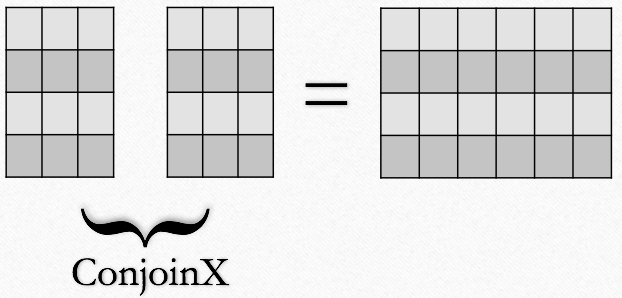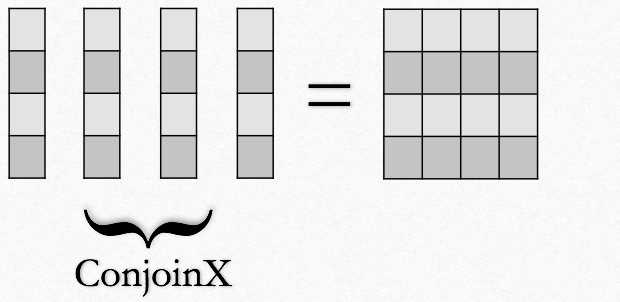$$\renewcommand\AA{\unicode{x212B}}$$

# ConjoinXRuns v1¶

## Summary¶

Joins the input workspaces horizontally by appending their columns.

## Properties¶

Name

Direction

Type

Default

Description

InputWorkspaces

Input

str list

Mandatory

The names of the input workspaces or workspace groups as a list. At least two point-data MatrixWorkspaces are required, having the same instrument, same number of spectra and units.

SampleLogAsXAxis

Input

string

The name of the numeric sample log to become the x-axis of the output. Empty by default, in which case the x-axis of the input workspaces are stitched. If specified, this will be the x-axis. It has to be numeric, in which case all the input workspaces must have only one point or numeric time series, in which case the number of elements in the series must match the number of points for each workspace.

OutputWorkspace

Output

Workspace

Mandatory

The output workspace.

SampleLogsTimeSeries

Input

string

A comma separated list of the sample logs to merge into a time series. The initial times are taken as the start times for the run. Sample logs must be numeric.

SampleLogsList

Input

string

A comma separated list of the sample logs to merge into a list.

SampleLogsWarn

Input

string

A comma separated list of the sample logs to generate a warning if different when merging.

SampleLogsWarnTolerances

Input

string

The tolerances for warning if sample logs are different. Can either be empty for a comparison of the strings, a single value for all warn sample logs, or a comma separated list of values (must be the same length as SampleLogsWarn).

SampleLogsFail

Input

string

The sample logs to fail if different when merging. If there is a difference the run is skipped.

SampleLogsFailTolerances

Input

string

The tolerances for failing if sample logs are different. Can either be empty for a comparison of the strings, a single value for all fail sample logs, or a comma separated list of values (must be the same length as SampleLogsFail).

SampleLogsSum

Input

string

A comma separated list of the sample logs to sum into a single entry. Sample logs must be numeric.

FailBehaviour

Input

string

Skip File

Choose whether to skip the workspace and continue, or stop and throw and error, when encountering a failure on merging. Allowed values: [‘Skip File’, ‘Stop’]

LinearizeAxis

Input

boolean

False

Choose to set a linear x-axis starting from 1, can be used only if the workspaces have common bins.

## Description¶

This algorithm joins the input workspaces into a single one by concatenating their spectra. The concatenation is done in the same order as in the input workspaces list. Consider using SortXAxis afterwards, if necessary. The instrument and the units are copied from the first workspace. The sample logs are also copied from the first input, but the behaviour can be controlled by the instrument parameter file (IPF), as described in MergeRuns but all parameter names must have the prefix conjoin_. Furthermore, that behaviour can be overridden by providing input to the relevant optional properties of the algorithm. This algorithm joins Dx values, if present.

## InputWorkspaces¶

This can be a mixed list of workspaces and workspace groups on AnalysisDataService (ADS), that will be flattened to a list of workspaces. MatrixWorkspaces representing point-data are required with:

• the same instrument

• the same number of histograms

• the same units

• each workspace must have the same amount of points per spectrum, however the x-axes may differ

## SampleLogAsXAxis¶

If specified, this log values will constitute the x-axis of the resulting workspace. The log must exist in all the input workspaces and must be numeric (int or double), in which case the input workspaces must contain single bin only, or numeric time series, in which case the length of the series must match the number of points.

## ConjoinX Operation¶

 Example case with 2 input workspaces with several points each. By default the original x-axes will be concatenated. If SampleLogAsXAxis is given, it has to be a numeric time series log with the same size as many points are in a row for each of the inputs. It will then make up the x-axis of the result.Example case with multiple input workspaces having a single point each. If SampleLogAsXAxis is given, it must be a numeric scalar (or a time series with a single value).## Usage¶

Example - ConjoinXRuns

# Create input workspaces
list = []
for i in range(3):
ws = "ws_{0}".format(i)
CreateSampleWorkspace(Function="One Peak", NumBanks=1, BankPixelWidth=2,
XMin=i*100, XMax=(i+1)*100, BinWidth=50,
Random=True, OutputWorkspace=ws)
ConvertToPointData(InputWorkspace=ws, OutputWorkspace=ws)
list.append(ws)

# Join the workspaces
out = ConjoinXRuns(list)

# Check the output
print("out has {0} bins with x-axis as: {1}, {2}, {3}, {4}, {5}, {6}".


Output:

out has 6 bins with x-axis as: 25.0, 75.0, 125.0, 175.0, 225.0, 275.0


Example - ConjoinXRuns with a numeric log

# Create input workspaces
list = []
for i in range(3):
ws = "ws_{0}".format(i)
CreateSampleWorkspace(Function="One Peak", NumBanks=1, BankPixelWidth=2,
XMin=i*100, XMax=(i+1)*100, BinWidth=100,
Random=True, OutputWorkspace=ws)
ConvertToPointData(InputWorkspace=ws, OutputWorkspace=ws)
list.append(ws)

# Join the workspaces
out = ConjoinXRuns(list, SampleLogAsXAxis='LOG')

# Check the output
print("out has {0} bins with x-axis as: {1}, {2}, {3}".


Output:

out has 3 bins with x-axis as: 0.0, 5.0, 10.0


Example - ConjoinXRuns with a numeric time series log

import datetime
# Create input workspaces
list = []
for i in range(3):
ws = "ws_{0}".format(i)
CreateSampleWorkspace(Function="One Peak", NumBanks=1, BankPixelWidth=2,
XMin=i*100, XMax=(i+1)*100, BinWidth=50,
Random=True, OutputWorkspace=ws)
ConvertToPointData(InputWorkspace=ws, OutputWorkspace=ws)

for j in range(2):

list.append(ws)

# Join the workspaces
out = ConjoinXRuns(list, SampleLogAsXAxis='LOG')

# Check the output
print("out has {0} bins with x-axis as: {1}, {2}, {3}, {4}, {5}, {6}".


Output:

out has 6 bins with x-axis as: 0.0, 0.25, 10.0, 10.25, 20.0, 20.25


Example - ConjoinXRuns to fail with a sample log forbidding to merge

 # Create input workspaces
list = []
for i in range(3):
ws = "ws_{0}".format(i)
CreateSampleWorkspace(Function="One Peak", NumBanks=1, BankPixelWidth=2,
XMin=i*100, XMax=(i+1)*100, BinWidth=50,
Random=True, OutputWorkspace=ws)
ConvertToPointData(InputWorkspace=ws, OutputWorkspace=ws)
list.append(ws)
try:
out = ConjoinXRuns(list, SampleLogsFail="Wavelength", SampleLogsFailTolerances="0.1", FailBehaviour="Stop")
except ValueError:
print("The differences in the wavelength of the inputs are more than the allowed tolerance")


Output:

The differences in the wavelength of the inputs are more than the allowed tolerance